## Example Questions

← Previous 1 3 4 5 6 7 8 9

### Example Question #1 : Polynomial Operations

What type of equation is the following?

(y + 2)(y + 4)(y + 1) = z

cubic

quartic

constant

linear

cubic

Explanation:

The degree of a polynomial is the highest exponent of the terms.

Degree 0 – constant

Degree 1 – linear

Degree 3 – cubic

Degree 4 – quartic

Multiply out the equation:

(y + 2)(y + 4)(y + 1) = z

(y2 + 2y + 4y + 8)(y + 1) = z

y3 + 2y2 + 4y2 + 8y + y2 + 2y + 4y + 8 = z

The highest exponent is y3;therefore the equation is a degree 3 cubic.

### Example Question #1 : Polynomials

Find the degree of the polynomial: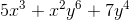Explanation:

The degree of a polynomial is determined by the term with the highest degree. In this case that is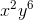, which has a degree of.

### Example Question #3 : Polynomial Operations

What is the degree of the following polynomial?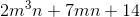Explanation:

The degree of a polynomial is determined by the term with the highest degree.  In this case, the first term,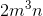, has the highest degree,. The degree of a term is calculated by adding the exponents of each variable in the term.

### Example Question #1 : Polynomials

What is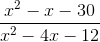equal to?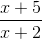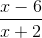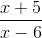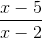Explanation:

1. Factor the numerator: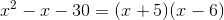2. Factor the denominator: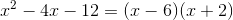3. Divide the factored numerator by the factored denominator: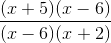You can cancel out the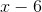from both the numerator and the denominator, leaving you with:### Example Question #5 : Polynomial Operations

Simplify: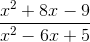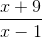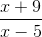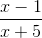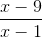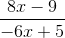Explanation:

In order to divide these polynomials, you need to first factor them.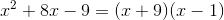and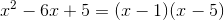Now, the expression becomes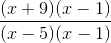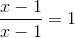so,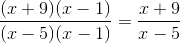### Example Question #6 : Polynomial Operations

Simplify the following division of polynomials: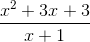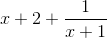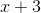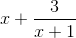It cannot be simplified any further.Explanation:

The leading term of the numerator is one exponent higher than the leading term of the denominator. Thus, we know the result of the division is going to be somewhere close to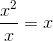. We can separate the fraction out like this: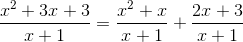The first term is easily seen to be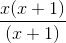, which is equal to. The second term can also be written out as: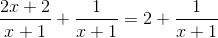and combining these, we get our final answer,### Example Question #7 : Polynomial Operations

Expand: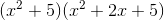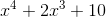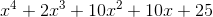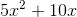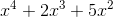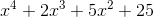Explanation:

Expand:Step 1: Use the distributive property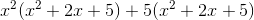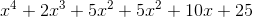Step 2: Combine like terms### Example Question #8 : Polynomial Operations

Two positive consecutive whole numbers that are even are both multiples of. The product of the two numbers is. What is the sum of the two integers?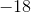Explanation:

The question provides two positive whole numbers that are each multiples of 6 and also 6 numbers apart. This may be translated into variables, where the first number may be represented by "x" and the second number may be represented as "x+6" given that it is 6 numbers greater than x.

The problem provides the information that the product of these two numbers is 72. Using the new definitions for the numbers, this may be represented as:

(x)(x+6) = 72

This provides an equation that multiplies two polynomials (one with one term, which is a monomial and one with two terms, which is a binomial) and an ability to solve for what x (the first of the two numbers) may be.

Using FOIL, the result is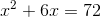. This may be rewritten as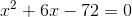, which will provide the value of x after factoring.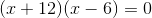, where (-6)(12) will provide the product of -72 and the sum of 12 and -6 will yield 6. The results indicate x as having two possible solutions: x=6 and x=-12. Returning back to the question, the goal is to find two positive numbers. This means that x=-12 is not a viable solution and that x=6 is. Now, revisiting the terms used to redefine the two numbers [x and x+6], x has been calculated. After substituting in the x value for the second term, the second number is 12 (6+6=12).

The final step of the problem is to solve for the sum of these two numbers:
6+12=18

### Example Question #9 : Polynomial Operations

Multiply these polynomials out and expand.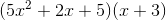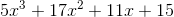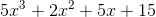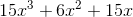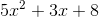Explanation:

The proper way to multiply polynomials is to essentially apply the distributive property continually.

Sois really the same as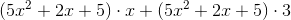, and so on.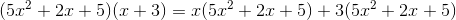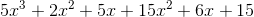Then, simply group together like terms to get the final answer:### Example Question #10 : Polynomial Operations

Simplify: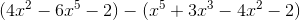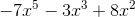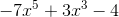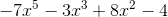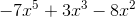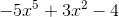Explanation:

When subtracting polynomials, it's helpful to remember that the "minus sign" gets distributed. It's as if the two polynomials are being added and a -1 is in front of the second polynomial.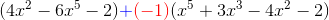This -1 will get multiplied to all the terms in the second polynomial that is being subtracted from the first, so it becomes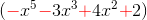. You may note that by multiplying by -1, every term in the polynomial switches its original sign. The problem then becomes: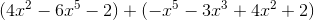From here, in order to simplify, because there's no equal sign, it can be deduced that we are not working toward a solution for x. The original problem is presented as an expression so an expression as an answer will be expected. In order to work towards a final simplified expression, like terms must be collected. This will provide the final answer.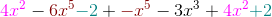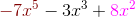← Previous 1 3 4 5 6 7 8 9